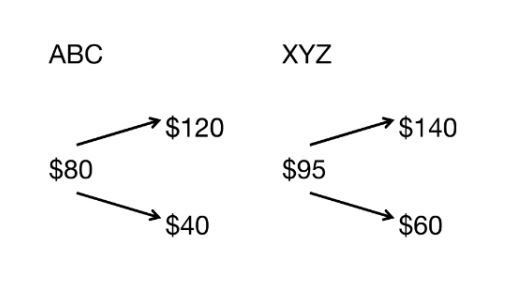Dec 11 / Exam Helper

# Which of the following positions creates a bull spread that has a zero payoff below a stock price of \$120, a positive payoff if the stock price exceeds \$120, and that yields its maximum payoff if the stock price is \$131 or greater on the expiration date of the contracts? Assume the current stock price is \$114, and that the risk-free rate is 3% per annum on a continuous basis. There may be more than one correct answer. Indicate all that are true.

a) a long call with a strike price of \$120 and a short call with a strike price of \$131, both expiring in one year

b) a long call with a strike price of \$114 and a short call with a strike price of \$131, both expiring in one year

c) a long call with a strike price of \$131 and a short call with a strike price of \$120, both expiring in one year

d) a long put with a strike price of \$120 and a short put with a strike price of \$131, both expiring in one year, and  \$11e-3%  invested in long position of one-year, zero-coupon bond

e) a long call with a strike price of \$120 and a short put with a strike price of \$131, both expiring in one year, and \$11e-3% invested in long position of one-year, zero-coupon bond

## Problem 3

The current forward price of one ounce of platinum for delivery one year from now is \$1'800. The current prices of some one-year European options on one ounce of platinum are shown below:

Price Information

Strike Price                                                                                Call Price                                                                Put Price

\$1'650                                                                              \$465                                                 \$330

\$1'920                                                                               \$???                                                 \$480

Based on these quoted prices, what is the price of the missing call option? Assume no cost of carry and no convenience yield.

Problem 4 (a)
Suppose you have two stocks, ABC and XYZ. ABC costs \$80 today, and will be worth \$120 in the up state of the world and \$40 in the down state. XYZ costs \$95 today and will be worth \$140 in the up state of the world and \$60 in the down state. The states that govern stocks ABC and XYZ are the same, so they have the same true and risk-neutral probabilities,(a) What is the risk-free interest rate? Write your answer in unit of percentage points.

b) What is the price today of the following portfolio: a put option on ABC with a strike of \$100, a call option on ABC with a strike of \$50, and a call option on XY7 with a strike of \$70.

#### Problem 5 (a)

The current price of a non-dividend paying stock is \$30. Use a two-step tree to value a European put option on the stock with a strike price of \$32 that expires in 6 months with the up node at each step equal to 1.1 times the price at the previous node, and the down node equal to 0.9 times the price at the previous node. Each step is 3 months; the risk free rate is 8% per annum on a continuous basis.

Q.a) What is the price of the put option?

Q.b) What is the price of the put option if the stock pays a dividend of \$3 in 3 months? Remember that the stock price immediately drops after dividend paid.

Q.c) Now, value an American call option with a strike price of \$25 on the same stock with identical price dynamics and interest rate as in part (b). Assume that if you exercise the option in 3 months you receive the dividend of \$3 paid on that date.